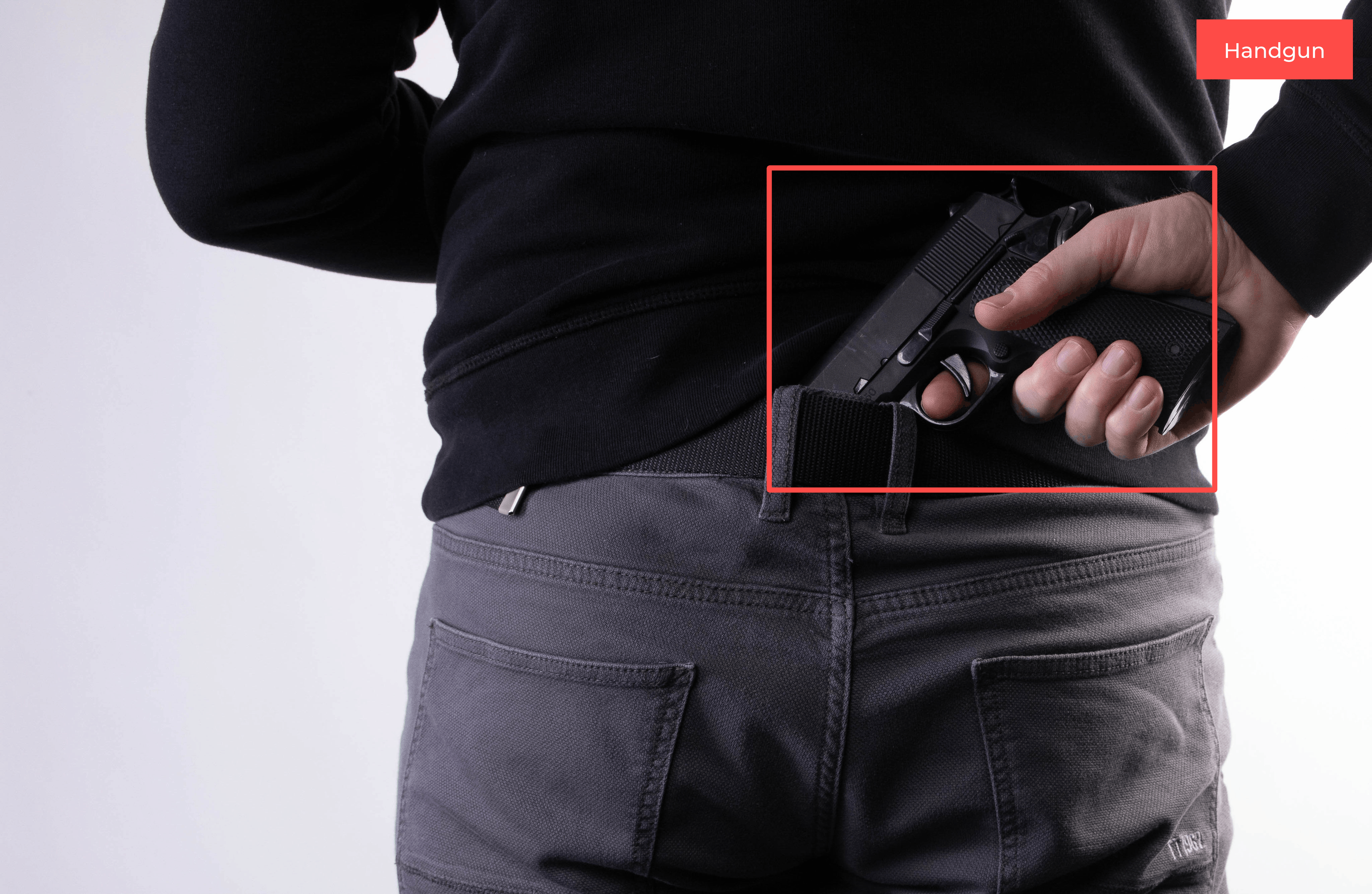#### HANDGUN DETECTOR (YOLOV4)On this website you can try the model with your own images and buy it if you are satisfied with its accuracy.

Model description:

This model detects handguns.

Number of classes: 1 (handgun)

Metrics:

detections_count = 1485, unique_truth_count = 659
class_id = 0, name = Handgun, ap = 93.92%        (TP = 590, FP = 72)
for conf_thresh = 0.25, precision = 0.89, recall = 0.90, F1-score = 0.89
for conf_thresh = 0.25, TP = 590, FP = 72, FN = 69, average IoU = 77.47 %
IoU threshold = 50 %, used Area-Under-Curve for each unique Recall
mean average precision (mAP@0.50) = 0.939233, or 93.92 %

System requirements:

Inference time using CPU: 300 ms (on HP Laptop 15-DA0042NH (Processor: Intel(R) Core(TM) i7-8550U CPU))